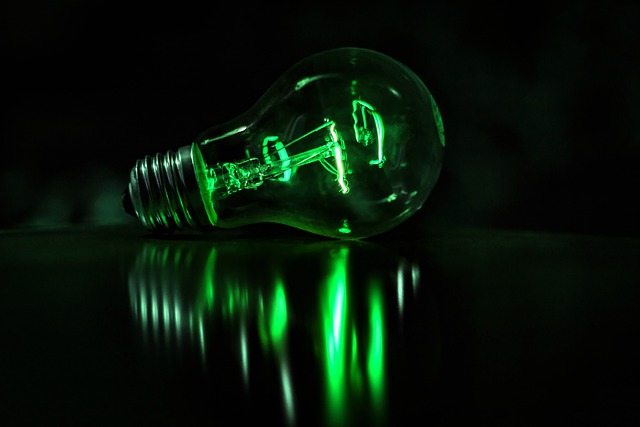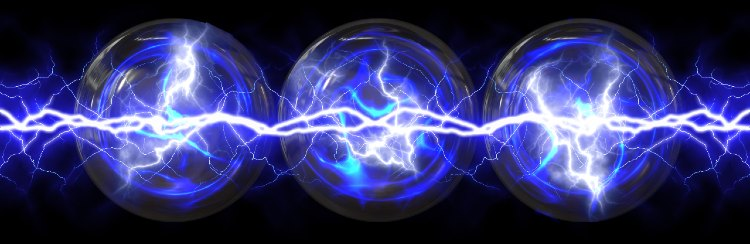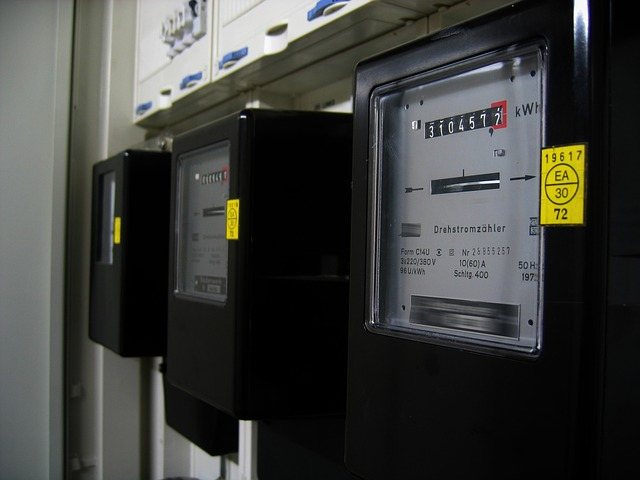Select Page

# A Complete Learning Guide

written by Stanley Udegbunam || Dec 16, 2020## WHAT IS ELECTRICAL ENERGY?

Electrical energy is energy resulting from the flow of electric charges.

It is energy generated whenever electrons move from one atom to another through a conducting medium.

The flow of electrons provides electric current that can be utilized to do work.

The faster the movement of the electron, the more energy they possess.

This will also magnify the intensity of the electric field.

## WHAT IS ELECTRIC FIELD?

Electric field is defined as the electric force per unit charge. It is the area surrounding a charged particle.

The direction of an electric field is always shown pointing in the direction a positive particle would travel if it was placed in the same field.

Therefore, the direction of the electric field indicates the direction of force it will exert on a positive test charge.

By convention, the electric field is radially outward from a positive charge and radially inward to a negative point charge.

The flow of electric charge through a conducting material produces electricity.## SOURCES OF ELECTRICAL ENERGY

Electrical energy is generated from different energy sources.

These sources are categorized into renewable energy sources and non-renewable energy sources.

Specific examples of electrical energy sources include:

• Coal
• Natural Gas
• Wind
• Hydro
• Nuclear Power
• Sun
• Biomass and
• Geothermal energy sources.

## EXAMPLES OF ELECTRICAL ENERGY

1. Alternating Current (A.C)

A.C is made available by electric power outlets in our homes and workplaces.

The electric power generated from these outlets is converted into other forms of energy like heat, light or sound energy.

A typical example is the electric pressing Iron. It changes the electric potential energy into heat energy for performing useful work.

1. Direct Current (D.C)

Electric energy is utilized in the form of direct current in small electronic components and majorly in devices that have circuit boards.

1. Batteries

They convert chemical energy into electrical energy through the use of one or more electrochemical cells.

1. Lightning

Lightning is an example of electric current.

It is the visible discharge of electricity that occurs when a region of a cloud acquires an excess electrical charge.

1. Energy generated by electric eels.

## USES OF ELECTRICAL ENERGY

Electrical energy is one of the most versatile and commonly used forms of energy in the world.

The use of electrical energy include:

• Electric energy is used to power energy-efficient vehicles like Tesla’s motors.
• Used for city power and illumination through billboards, street lights and traffic lights.
• Electrical energy is used in hospitals and medical centers for X-ray machines and life support machines.
• It is generally used to power electric devices and household appliances like television, vacuum cleaner, electric cooker, washing machine etc.
• Electrical energy is present in living cells, tissues and organisms in the form of bioelectricity.electrical energy is used to power homes and cities

## FORMULA FOR ELECTRICAL ENERGY

The formula for electrical energy is:

Electrical energy = Power x Time

Power is expressed in watts, and

time is expressed in seconds.

Therefore, the unit of Electrical energy is Joules or watt-seconds.

## CALCULATIONS ON ELECTRICAL ENERGY

A 2500-watt electric cooker is used to prepare meal for a duration of one hour (3600 seconds),

Calculate the energy expended.

Solution

Energy = P x T = 2500 x 3600 = 9,000 J

Energy = 9,000,000 joules = 9,000 kilojoules.

A kilowatt-hour is the amount of electrical energy that is used by a 1,000 watt appliance within the duration of one hour.

1kwh = 1000 w x 3600 seconds = 3,600,000 joules;

3,600,000 joules = 3,600 kilojoules;

Therefore,

1kwh = 3,600 kilojoules.

The electric cooker discussed above utilized an electrical energy of 9,000 KJ.

Expressing this in terms of KWH will yield 9,000/3,600 = 2.5 Kwh.

Therefore, 9,000 KJ = 2.5 Kwh

## Why do Utility Companies sell Power in Kilowatt Hour (KWH)?

This is because Kilowatt Hour is a more convenient unit to measure power compared to other energy units.

Electrical devices have different consumption rates, and measuring the total energy consumption in Joules will yield values that are too high to be estimated on a bill.

Since these devices consume power over a given time interval, Kilowatt-hour then becomes a better unit for sales.

Kilowatt-hour accounts for the total energy consumption of a household in time intervals of one hour.

For instance, the electric cooker from our calculation above consumes 9,000 Joules in 1 hour, although it’s slightly fictional, it’s not far fetched from what is obtainable in a real-life scenario.

Now, imagine if we calculate the energy consumed by multiple electrical appliances present in a house over the course of a day (24 hours).

I guess we’ll exceed the screen allocation for numerals on the calculator.

Therefore kilowatt-hour (Kwh) is a better unit not just for power sales but also for calculations and energy analysis.electricity meters